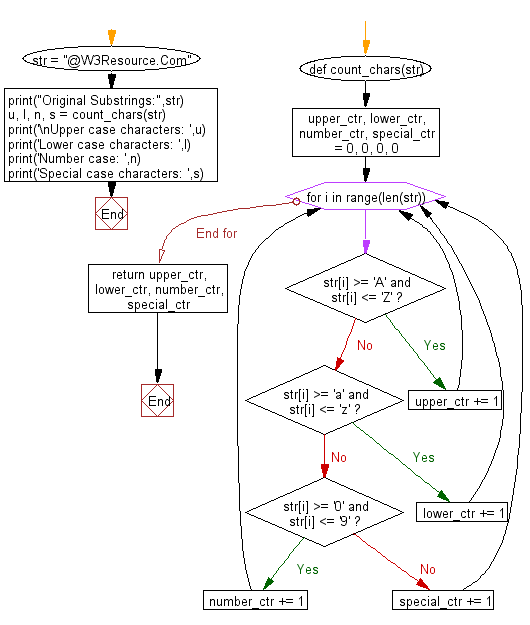﻿ Python: Count Uppercase, Lowercase, special character and numeric values in a given string - w3resource# Python: Count Uppercase, Lowercase, special character and numeric values in a given string

## Python String: Exercise-73 with Solution

Write a Python program to count Uppercase, Lowercase, special character and numeric values in a given string.

Sample Solution:-

Python Code:

``````def count_chars(str):
upper_ctr, lower_ctr, number_ctr, special_ctr = 0, 0, 0, 0
for i in range(len(str)):
if str[i] >= 'A' and str[i] <= 'Z': upper_ctr += 1
elif str[i] >= 'a' and str[i] <= 'z': lower_ctr += 1
elif str[i] >= '0' and str[i] <= '9': number_ctr += 1
else: special_ctr += 1
return upper_ctr, lower_ctr, number_ctr, special_ctr

str = "@W3Resource.Com"
print("Original Substrings:",str)
u, l, n, s = count_chars(str)
print('\nUpper case characters: ',u)
print('Lower case characters: ',l)
print('Number case: ',n)
print('Special case characters: ',s)
```
```

Sample Output:

```Original Substrings: @W3Resource.Com

Upper case characters:  3
Lower case characters:  9
Number case:  1
Special case characters:  2
```

Pictorial Presentation:Flowchart:## Visualize Python code execution:

The following tool visualize what the computer is doing step-by-step as it executes the said program:

Python Code Editor:

Have another way to solve this solution? Contribute your code (and comments) through Disqus.

What is the difficulty level of this exercise?

Test your Programming skills with w3resource's quiz.

﻿

## Python: Tips of the Day

Check if a given key already exists in a dictionary:

In is the intended way to test for the existence of a key in a dict.

```d = {"key1": 10, "key2": 23}

if "key1" in d:
print("this will execute")

if "nonexistent key" in d:
print("this will not")
```

If you wanted a default, you can always use dict.get():

```d = dict()

for i in range(100):
key = i % 10
d[key] = d.get(key, 0) + 1
```

and if you wanted to always ensure a default value for any key you can either use dict.setdefault() repeatedly or defaultdict from the collections module, like so:

```from collections import defaultdict

d = defaultdict(int)

for i in range(100):
d[i % 10] += 1
```

but in general, the in keyword is the best way to do it.

Ref: https://bit.ly/2XPMRyz